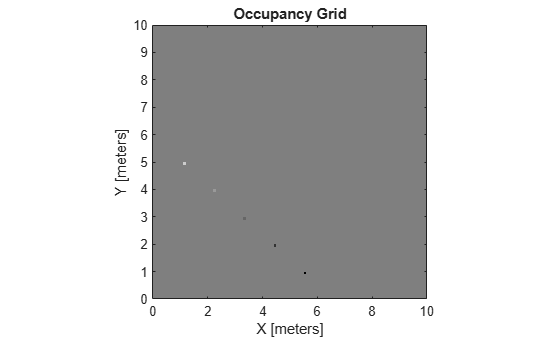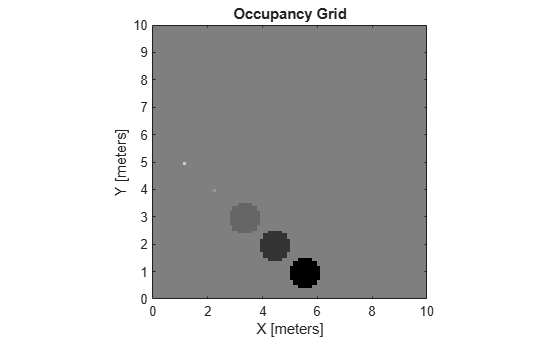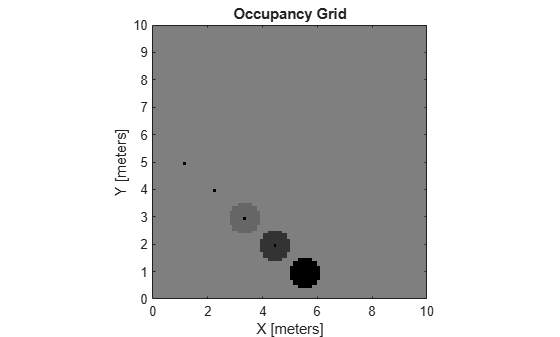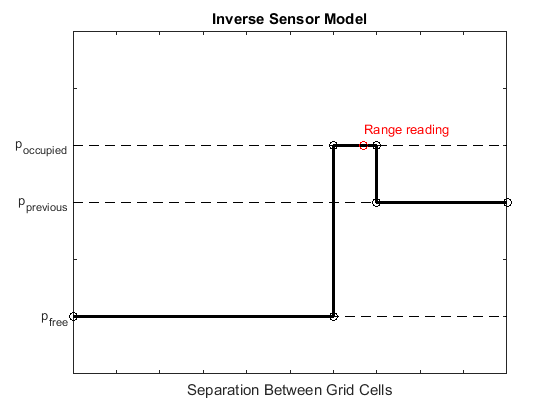# updateOccupancy

Integrate probability observations at locations

## Syntax

``updateOccupancy(map,xy,obs)``
``updateOccupancy(map,ij,occval,'grid')``

## Description

example

````updateOccupancy(map,xy,obs)` probabilistically integrates the observation values, `obs`, to each coordinate specified in `xy`. Observation values are determined based on the Inverse Sensor Model.```
````updateOccupancy(map,ij,occval,'grid')` probabilistically integrates the occupancy values, `occval`, to the specified grid locations, `ij`, instead of world coordinates.```

## Examples

collapse all

Create a 10 m-by-10 m empty map.

`map = occupancyMap(10,10,10);`

Update the occupancy of world locations with specific probability values and display the map.

```x = [1.2; 2.3; 3.4; 4.5; 5.6]; y = [5.0; 4.0; 3.0; 2.0; 1.0]; pvalues = [0.2 0.4 0.6 0.8 1]; updateOccupancy(map,[x y],pvalues) figure show(map)```Inflate occupied areas by a radius of 0.5 m. Larger occupancy values overwrite the smaller values.

```inflate(map,0.5) figure show(map)```Get grid locations from world locations.

`ij = world2grid(map,[x y]);`

Set grid locations to occupied locations.

```setOccupancy(map,ij,ones(5,1),'grid') figure show(map)```## Input Arguments

collapse all

Map representation, specified as a `occupancyMap` object. This object represents the environment of the vehicle. The object contains a matrix grid with values representing the probability of the occupancy of that cell. Values close to 1 represent a high probability that the cell contains an obstacle. Values close to 0 represent a high probability that the cell is not occupied and obstacle free.

World coordinates, specified as an n-by-2 vertical matrix of ```[x y]``` pairs, where n is the number of world coordinates.

Data Types: `double`

Grid positions, specified as an n-by-2 matrix of ```[i j]``` pairs in ```[rows cols]``` format, where n is the number of grid positions.

Data Types: `double`

Probability observation values, specified as a scalar or an n-by-1 column vector the same size as either `xy` or `ij`.

`obs` values can be any value from 0 to 1, but if `obs` is a logical vector, the default observation values of 0.7 (`true`) and 0.4 (`false`) are used. These values correlate to the inverse sensor model for ray casting. If `obs` is a numeric or a logical scalar, the value is applied to all coordinates in `xy` or `ij`.

Probability occupancy values, specified as a scalar or a column vector the same size as either `xy` or `ij`. A scalar input is applied to all coordinates in either `xy` or `ij`.

Values close to 0 represent a high probability that the cell is not occupied and obstacle free.

collapse all

### Inverse Sensor Model

The inverse sensor model determines how values are set along a ray from a range sensor reading to the obstacles in the map. `NaN` range values are ignored. Range values greater than `maxrange` are not updated.Grid locations that contain range readings are updated with the occupied probability. Locations before the reading are updated with the free probability. All locations after the reading are not updated.